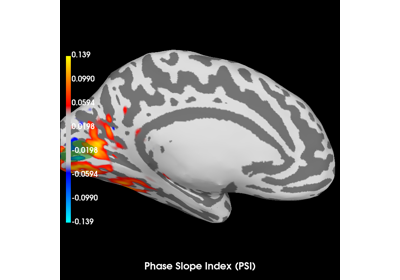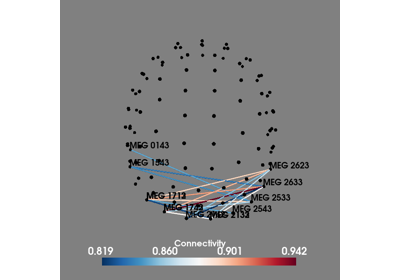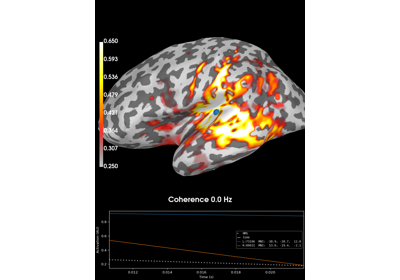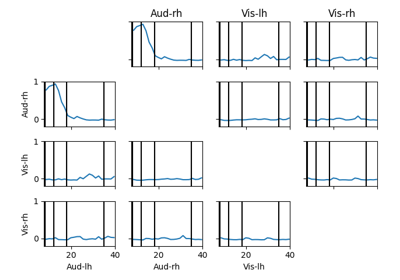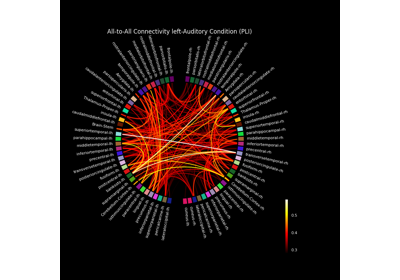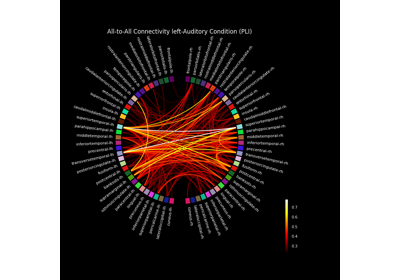# mne_connectivity.SpectralConnectivity¶

class mne_connectivity.SpectralConnectivity(data, freqs, n_nodes, names=None, indices='all', method=None, spec_method=None, n_epochs_used=None, **kwargs)[source]

Spectral connectivity class.

This class stores connectivity data that varies over frequencies. The underlying data is an array of shape (n_connections, n_freqs), or (n_nodes, n_nodes, n_freqs).

Parameters

data : np.ndarray ([epochs], n_estimated_nodes, [freqs], [times])

The connectivity data that is a raveled array of `(n_estimated_nodes, ...)` shape. The `n_estimated_nodes` is equal to `n_nodes_in * n_nodes_out` if one is computing the full connectivity, or a subset of nodes equal to the length of `indices` passed in.

freqs : list | np.ndarray

The frequencies at which the connectivity data is computed over. If the frequencies are “frequency bands” (i.e. gamma band), then these are the median of those bands.

n_nodes : int

The number of nodes in the dataset used to compute connectivity. This should be equal to the number of signals in the original dataset.

names : list | np.ndarray | None

The names of the nodes of the dataset used to compute connectivity. If ‘None’ (default), then names will be a list of integers from 0 to `n_nodes`. If a list of names, then it must be equal in length to `n_nodes`.

indices : tuple of arrays | str | None

The indices of relevant connectivity data. If `'all'` (default), then data is connectivity between all nodes. If `'symmetric'`, then data is symmetric connectivity between all nodes. If a tuple, then the first list represents the “in nodes”, and the second list represents the “out nodes”. See “Notes” for more information.

method : str, optional

The method name used to compute connectivity.

spec_method : str, optional

The type of method used to compute spectral analysis, by default None.

n_epochs_used : int, optional

The number of epochs used in the computation of connectivity, by default None.

Attributes

`attrs`

Xarray attributes of connectivity.

`coords`

The coordinates of the xarray data.

`dims`

The dimensions of the xarray data.

`freqs`

The frequency points of the connectivity data.

`indices`

Indices of connectivity data.

`method`

The method used to compute connectivity.

`n_epochs_used`

Number of epochs used in computation of connectivity.

`n_nodes`

The number of nodes in the original dataset.

`names`

Node names.

`shape`

Shape of raveled connectivity.

`xarray`

Xarray of the connectivity data.

Methods

 `get_data`([output]) Get connectivity data as a numpy array. `plot_circle`(**kwargs) Visualize connectivity as a circular graph. `predict`(data) Predict samples on actual data. `rename_nodes`(mapping) Rename nodes. `save`(fname) Save connectivity data to disk. `simulate`(n_samples[, noise_func, random_state]) Simulate vector autoregressive (VAR) model.
property attrs

Xarray attributes of connectivity.

See `xarray`’s `attrs`.

property coords

The coordinates of the xarray data.

property dims

The dimensions of the xarray data.

property freqs

The frequency points of the connectivity data.

If these are computed over a frequency band, it will be the median frequency of the frequency band.

get_data(output='compact')

Get connectivity data as a numpy array.

Parameters

output : str, optional

How to format the output, by default ‘raveled’, which will represent each connectivity matrix as a `(n_nodes_in * n_nodes_out,)` list. If ‘dense’, then will return each connectivity matrix as a 2D array. If ‘compact’ (default) then will return ‘raveled’ if `indices` were defined as a list of tuples, or `dense` if indices is ‘all’.

Returns

data : np.ndarray

The output connectivity data.

property indices

Indices of connectivity data.

Returns

indices : str | tuple of lists

Either ‘all’ for all-to-all connectivity, ‘symmetric’ for symmetric all-to-all connectivity, or a tuple of lists representing the node-to-nodes that connectivity was computed.

property method

The method used to compute connectivity.

property n_epochs_used

Number of epochs used in computation of connectivity.

Can be ‘None’, if there was no epochs used.

property n_nodes

The number of nodes in the original dataset.

Even if `indices` defines a subset of nodes that were computed, this should be the total number of nodes in the original dataset.

property names

Node names.

plot_circle(**kwargs)

Visualize connectivity as a circular graph.

Parameters

node_names : list of str

Node names. The order corresponds to the order in con.

indices : tuple of array | None

Two arrays with indices of connections for which the connections strengths are defined in con. Only needed if con is a 1D array.

n_lines : int | None

If not None, only the n_lines strongest connections (strength=abs(con)) are drawn.

node_angles : array, shape (n_node_names,) | None

Array with node positions in degrees. If None, the nodes are equally spaced on the circle. See mne.viz.circular_layout.

node_width : float | None

Width of each node in degrees. If None, the minimum angle between any two nodes is used as the width.

node_colors : list of tuple | list of str

List with the color to use for each node. If fewer colors than nodes are provided, the colors will be repeated. Any color supported by matplotlib can be used, e.g., RGBA tuples, named colors.

facecolor : str

Color to use for background. See matplotlib.colors.

textcolor : str

Color to use for text. See matplotlib.colors.

node_edgecolor : str

Color to use for lines around nodes. See matplotlib.colors.

linewidth : float

Line width to use for connections.

colormap : str | instance of matplotlib.colors.LinearSegmentedColormap

Colormap to use for coloring the connections.

vmin : float | None

Minimum value for colormap. If None, it is determined automatically.

vmax : float | None

Maximum value for colormap. If None, it is determined automatically.

colorbar : bool

Display a colorbar or not.

title : str

The figure title.

colorbar_size : float

Size of the colorbar.

colorbar_pos : tuple, shape (2,)

Position of the colorbar.

fontsize_title : int

Font size to use for title.

fontsize_names : int

Font size to use for node names.

fontsize_colorbar : int

Font size to use for colorbar.

Space to add around figure to accommodate long labels.

fig : None | instance of matplotlib.figure.Figure

The figure to use. If None, a new figure with the specified background color will be created.

subplot : int | tuple, shape (3,)

Location of the subplot when creating figures with multiple plots. E.g. 121 or (1, 2, 1) for 1 row, 2 columns, plot 1. See matplotlib.pyplot.subplot.

interactive : bool

When enabled, left-click on a node to show only connections to that node. Right-click shows all connections.

node_linewidth : float

Line with for nodes.

show : bool

Show figure if True.

Returns

fig : instance of matplotlib.figure.Figure

The figure handle.

axes : instance of matplotlib.projections.polar.PolarAxes

The subplot handle.

Notes

This code is based on a circle graph example by Nicolas P. Rougier

By default, `matplotlib.pyplot.savefig()` does not take `facecolor` into account when saving, even if set when a figure is generated. This can be addressed via, e.g.:

```>>> fig.savefig(fname_fig, facecolor='black')
```

If `facecolor` is not set via `matplotlib.pyplot.savefig()`, the figure labels, title, and legend may be cut off in the output figure.

predict(data)

Predict samples on actual data.

The result of this function is used for calculating the residuals.

Parameters

data : array

Epoched or continuous data set. Has shape (n_epochs, n_signals, n_times) or (n_signals, n_times).

Returns

predicted : array

Data as predicted by the VAR model of shape same as `data`.

Notes

Residuals are obtained by r = x - var.predict(x).

To compute residual covariances:

```# compute the covariance of the residuals
# row are observations, columns are variables
t = residuals.shape
sampled_residuals = np.concatenate(
np.split(residuals[:, :, model_order:], t, 0),
axis=2
).squeeze(0)
rescov = np.cov(sampled_residuals)
```
rename_nodes(mapping)

Rename nodes.

Parameters

mapping : dict

Mapping from original node names (keys) to new node names (values).

save(fname)

Save connectivity data to disk.

Parameters

fname : str | pathlib.Path

The filepath to save the data. Data is saved as netCDF files (`.nc` extension).

property shape

Shape of raveled connectivity.

simulate(n_samples, noise_func=None, random_state=None)

Simulate vector autoregressive (VAR) model.

This function generates data from the VAR model.

Parameters

n_samples : int

Number of samples to generate.

noisefunc : func, optional

This function is used to create the generating noise process. If set to None, Gaussian white noise with zero mean and unit variance is used.

Returns

data : array, shape (n_samples, n_channels)

Generated data.

property xarray

Xarray of the connectivity data.

## Examples using `mne_connectivity.SpectralConnectivity`¶